# Phase Portraits of Complex Functions with the R Package viscomplexr

## Introduction

The R package viscomplexr has been written as a visualization tool for complex functions. More precisely, it provides functionality for making phase portraits of such functions. The method, sometimes called domain coloring, exists in many sub-varieties. However, from the author’s point of view, the style proposed by E. Wegert in his book Visual Complex Functions (Wegert 2012) comes with a particular clarity and a special aesthetic appeal at the same time. Therefore, this package closely follows Wegert’s conventions. Conceptually, the package is intended for being used inside the framework of R’s base graphics, i.e. users of this package can freely utilize all features of base graphics for obtaining an optimum result, be it for scientific or artistic purposes. This vignette is not at all an introduction to function theory or an exhaustive treatment of what can be done with phase portraits - I recommend Wegert’s book for an ideal combination of both; the purpose of this vignette is in fact to make the reader acquainted with the technical features the package provides in a step-by-step process.

Due to the size restriction of CRAN packages, the number of illustrations in this vignette is kept to a minimum. Readers are encouraged to run all code examples shown below (and hopefully enjoy what they see), but especially those where we explicitly invite them to do so.

## Using the function phasePortrait

### Visualizing the complex plane

The package does not contain many functions, but provides a very versatile workhorse called phasePortrait. We will explore some of its key features now. Let us first consider a function that maps a complex number $$z \in \mathbb{C}$$ on itself, i.e. $$f(z)=z$$. After attaching the package with library(viscomplexr), a phase portrait of this function is obtained very easily with:

phasePortrait("z", xlim = c(-8.5, 8.5), ylim = c(-8.5, 8.5),
xlab = "real", ylab = "imaginary", main = "f(z) = z",
nCores = 2) # Probably not required on your machine (see below)

# Note the argument 'nCores' which determines the number of parallel processes to
# be used. Setting nCores = 2 has been done here and in all subsequent
# examples as CRAN checks do not allow more parallel processes.
# For normal work, we recommend not to define nCores at all which will make
# phasePortrait use all available cores on your machine.

# The progress messages phasePortrait is writing to the console can be
# suppressed by including 'verbose = FALSE' in the call (see documentation).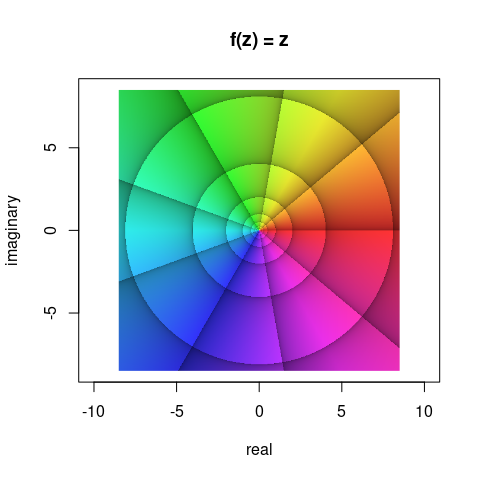Phase portrait of the function $$f(z)=z$$ in the window $$\left|\Re(z)\right| < 8.5$$ and $$\left|\Im(z)\right| < 8.5$$.

Such a phase portrait is based on the polar representation of complex numbers. Any complex number $$z$$ can be written as $$z=r\cdot\mathrm{e}^{\mathrm{i}\varphi}$$ or equivalently $$z=r\cdot(\cos\varphi+\mathrm{i}\cdot\sin\varphi)$$, whereby $$r$$ is the modulus and the angle $$\varphi$$ is the argument. The argument, also called the phase angle, is the angle in the origin of the complex number plane between the real axis and the position vector of the number in counter-clockwise orientation. The main feature of a phase portrait is to translate the argument into a color. In addition, there are options for visualizing the modulus or, more precisely, its relative change.

The translation of the phase angle $$\varphi$$ into a color follows the hsv color model, where radian values of $$\varphi=0+k\cdot2\pi$$, $$\varphi=\frac{2\pi}{3}+k\cdot2\pi$$, and $$\varphi=\frac{4\pi}{3}+k\cdot2\pi$$ with $$k\in\mathbb{Z}$$ translate into the colors red, green, and blue, respectively, with a continuous transition of colors with values between. As all numbers with the same argument $$\varphi$$ obtain the same color, the numbers of the complex plane as visualized in the Figure above are colored along the chromatic cycle. In order to add visual structure, argument values of $$\varphi=\frac{2\pi}{9}$$, i.e. $$40°$$ and their integer multiples are emphasized by black lines. Note that each of these lines follows exactly one color. Moreover, the zones between two neighboring arguments $$\varphi_1=k\cdot\frac{2\pi}{9}$$ and $$\varphi_2=(k+1)\cdot\frac{2\pi}{9}$$ with $$k\in\mathbb{Z}$$ are shaded in a way that the brightness of the colors inside one such zone increases with increasing $$\varphi$$, i.e. in counterclockwise sense of rotation.

The other lines visible in the figure above relate to the modulus $$r$$. One such line follows the same value of $$r$$; it is thus obvious that each of these iso-modulus lines must form a concentric circle on the complex number plane (see the figure above). The distance between neighboring iso-modulus lines is chosen so that it always indicates the same relative change. For reasons to talk about later (see also Wegert 2012), the default setting of the function phasePortrait is a relative change of $$b=\mathrm{e}^{2\pi/9}$$ which is very close to $$2$$. Thus, with a grain of salt, the modulus of the complex numbers doubles or halves when moving from one iso-modulus line to the other. In the phase portrait, the zones between two adjacent iso-modulus lines are shaded in a way that the colors inside such a zone become brighter in the direction of increasing modulus. The lines themselves are located at the moduli $$r=b^k$$, with $$k\in\mathbb{Z}$$. This is nicely visible in the phase portrait above, where the outmost circular iso-modulus line indicates (approximately, as $$b$$ is not exactly $$2$$) $$r=2^3=8$$. Moving inwards, the following iso-modulus lines are at (approximately) $$r=2^2=4$$, $$r=2^1=2$$, $$r=2^0=1$$, $$r=2^{-1}=\frac{1}{2}$$, $$r=2^{-2}=\frac{1}{4}$$, etc. Obviously, as the modulus of the numbers on the complex plane is their distance from the origin, the width of the concentric rings formed by adjacent iso-modulus lines approximately doubles from ring to ring when moving outwards.

### Visual structuring - the argument pType

When working with the function phasePortrait, it might not always be desirable to display all of these reference lines and zonings. The argument pType allows for four different options as illustrated in the next example:

# divide graphics device into four regions and adjust plot margins
op <- par(mfrow = c(2, 2),
mar   = c(0.25, 0.55, 1.10, 0.25))
# plot four phase portraits with different choices of pType
phasePortrait("z", xlim = c(-8.5, 8.5), ylim = c(-8.5, 8.5), pType = "p",
main = "pType = 'p'",   axes = FALSE, nCores = 2)
phasePortrait("z", xlim = c(-8.5, 8.5), ylim = c(-8.5, 8.5), pType = "pa",
main = "pType = 'pa'",  axes = FALSE, nCores = 2)
phasePortrait("z", xlim = c(-8.5, 8.5), ylim = c(-8.5, 8.5), pType = "pm",
main = "pType = 'pm'",  axes = FALSE, nCores = 2)
phasePortrait("z", xlim = c(-8.5, 8.5), ylim = c(-8.5, 8.5), pType = "pma",
main = "pType = 'pma'", axes = FALSE, nCores = 2)
par(op) # reset the graphics parameters to their previous values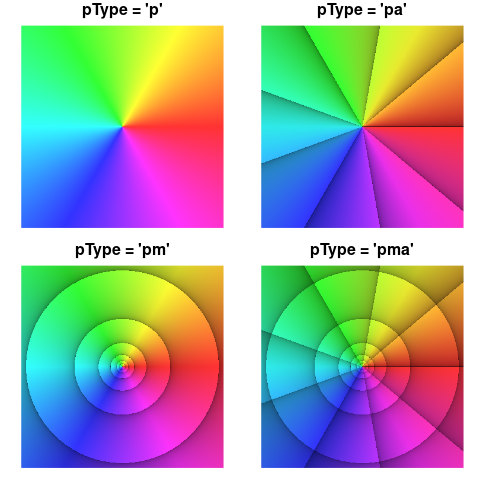Different options for including reference lines with the argument pType.

As evident from the figure above, setting ptype to ‘p’ displays a phase portrait in the literal sense, i.e. only the phase of the complex numbers is displayed and nothing else. The option ‘pa’ adds reference lines for the argument, the option ‘pm’ adds iso-modulus lines, and the (default) option ‘pma’ adds both. In addition to these options, the example shows phasePortrait in combination with R’s base graphics. The first and the last line of the code chunk set and reset global graphics parameters, and inside the calls to phasePortrait, we use the arguments main (diagram title) and axes which are generic plot arguments.

### Visual structuring - the arguments pi2Div and logBase

For demonstrating options to adjust the density of the argument and modulus reference lines, consider the rational function $f(z)=\frac{(3+2\mathrm{i}+z)(-5+5\mathrm{i}+z)}{(-2-2\mathrm{i}+z)^2}$ Evidently, this function has two zeroes, $$z_1=-3-2\mathrm{i}$$, and $$z_2=5-5\mathrm{i}$$. It also has a second order pole at $$z_3=2+2\mathrm{i}$$. We make a phase portrait of this function over the same cutout of the complex plane as we did in the figures above. When calling phasePortrait with such simple functions, it is most convenient to define them as as a quoted character string in R syntax containing the variable $$z$$. Run the code below for displaying the phase portrait (active 7" x 7" screen graphics device suggested, e.g. x11()).

op <- par(mar = c(5.1, 4.1, 2.1, 2.1), cex = 0.8) # adjust plot margins
# and general text size
phasePortrait("(3+2i+z)*(-5+5i+z)/(-2-2i+z)^2",
xlim = c(-8.5, 8.5), ylim = c(-8.5, 8.5),
xlab = "real", ylab = "imaginary",
nCores = 2) # Increase or leave out for higher performance
par(op) # reset the graphics parameters to their previous values

The resulting figure nicely displays the function’s two zeroes and the pole. Note that all colors meet in zeroes and poles. Around zeroes, the colors cycle counterclockwise in the order red, green, blue, while this order is reversed around poles. For $$n$$th order ($$n\in\mathbb{N}$$) zeroes and poles, the cycle is passed through $$n$$ times. I recommend to check this out with examples of your own.

Now, suppose we want to change the density of the reference lines for the phase angle $$\varphi$$. This can be done by way of the argument pi2Div. For usual applications, pi2Div should be a natural number $$n\:(n\in\mathbb{N})$$. It defines the angle $$\Delta\varphi$$ between two adjacent reference lines as a fraction of the round angle, i.e. $$\Delta\varphi=\frac{2\pi}{n}$$. The default value of pi2Div is 9, i.e. $$\Delta\varphi=\frac{2\pi}{9}=40°$$. Let’s plot our function in three flavors of pi2Div, namely, 6, 9 (the default), and 18, resulting in $$\Delta\varphi$$ values of $$\frac{\pi}{3}=60°$$, $$\frac{2\pi}{9}=40°$$, and $$\frac{\pi}{9}=20°$$. In order to suppress the iso-modulus lines and display the argument reference lines only, we are using pType = "pa". Visualize this by running the code below (active 7" x 2.8" screen graphics device suggested, e.g. x11(width = 7, height = 2.8)).

# divide graphics device into three regions and adjust plot margins
op <- par(mfrow = c(1, 3), mar = c(0.2, 0.2, 0.4, 0.2))
for(n in c(6, 9, 18)) {
phasePortrait("(3+2i+z)*(-5+5i+z)/(-2-2i+z)^2", xlim = c(-8.5, 8.5), ylim = c(-8.5, 8.5),
pi2Div = n, pType = "pa", axes = FALSE, nCores = 2)
# separate title call (R base graphics) for nicer line adjustment, just cosmetics
title(paste("pi2Div =", n), line = -1.2)
}
par(op) # reset graphics parameters to previous values

So far, this is exactly, what had to be expected. But see what happens when we choose the default pType, "pma" which also adds modulus reference lines:

# divide graphics device into three regions and adjust plot margins
op <- par(mfrow = c(1, 3), mar = c(0.2, 0.2, 0.4, 0.2))
for(n in c(6, 9, 18)) {
phasePortrait("(3+2i+z)*(-5+5i+z)/(-2-2i+z)^2", xlim = c(-8.5, 8.5), ylim = c(-8.5, 8.5),
pi2Div = n, pType = "pma", axes = FALSE, nCores = 2)
# separate title call (R base graphics) for nicer line adjustment, just cosmetics
title(paste("pi2Div =", n), line = -1.2)
}
par(op) # reset graphics parameters to previous values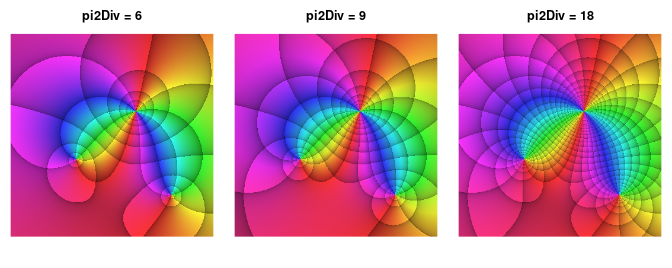The function $$f(z)=\frac{(3+2\mathrm{i}+z)(-5+5\mathrm{i}+z)}{(-2-2\mathrm{i}+z)^2}$$ portrayed with three different settings of pi2Div and pType = "pma".

Evidently, the choice of pi2Div has influenced the density of the iso-modulus lines. This is because, by default, the parameter logBase, which controls how dense the iso-modulus lines are arranged, is linked to pi2Div. As stated above, pi2Div is usually a natural number $$n\:(n \in\mathbb{N})$$, and logBase is the real number $$b\:(b\in\mathbb{R})$$ which defines the moduli $$r=b^k\:(k\in\mathbb{Z})$$ where the reference lines are drawn. When $$n$$ is given, the default definition of $$b$$ is $$b=\mathrm{e}^{2\pi/n}$$. In the default case, $$n=9$$, this results in $$b\approx2.009994$$. Thus, by default, moving from one iso-modulus line to the adjacent one means almost exactly doubling or halving the modulus, depending on the direction. For the other two cases $$n=6$$ and $$n=18$$, the resulting values for $$b$$ are $$b\approx2.85$$ and $$b\approx1.42$$, the latter obviously being the square root of $$\mathrm{e}^{2\pi/9}$$. For $$n=9$$, the modulus (approximately) doubles or halves when traversing two adjacent iso-modulus lines.

Before we demonstrate the special property of this linkage between $$n$$ and $$b$$, i.e. between pi2Div and logBase, we shortly show, that they can be decoupled in phasePortrait without any complication. In the following example, we want to define the density of the iso-modulus lines in a way that the modulus triples when traversing three zones in the direction of ascending moduli. Clearly, this requires to define logBase as $$b=\sqrt{3}\approx1.44$$. Thus, when moving from one iso-modulus line to the next higher one, the modulus has increased by a factor of about $$1.4$$. One line further, it has about doubled ($${\sqrt{3}}^{2}\approx2.08$$), and another line further it has exactly tripled. While varying pi2Div exactly as in the previous example, we now keep logBase constant at $$\sqrt{3}$$. Run the code below for visualizing this (active 7" x 2.8" screen graphics device suggested, e.g. x11(width = 7, height = 2.8)).

# divide graphics device into three regions and adjust plot margins
op <- par(mfrow = c(1, 3), mar = c(0.2, 0.2, 0.4, 0.2))
for(n in c(6, 9, 18)) {
phasePortrait("(3+2i+z)*(-5+5i+z)/(-2-2i+z)^2", xlim = c(-8.5, 8.5), ylim = c(-8.5, 8.5),
pi2Div = n, logBase = sqrt(3), pType = "pma", axes = FALSE, nCores = 2)
# separate title call (R base graphics) for nicer line adjustment, just cosmetics
title(paste("pi2Div = ", n, ", logBase = 3^(1/3)", sep = ""), line = -1.2)
}
par(op) # reset graphics parameters to previous values

In order to understand why by default the parameters pi2Div and logBase are linked as described above, we consider the exponential function $$f(z)=\mathrm{e}^z$$. We can write $$z=r\cdot(\cos\varphi+\mathrm{i}\cdot\sin\varphi)$$ and thus $$f(z)=\mathrm{e}^{r\cdot(\cos\varphi+\mathrm{i}\cdot\sin\varphi)}$$ or $$w=f(z)=\mathrm{e}^{r\cdot\cos\varphi}\cdot\mathrm{e}^{\mathrm{i}\cdot r\cdot\sin\varphi}$$. The modulus of $$w$$ is $$\mathrm{e}^{r\cdot\cos\varphi}$$ and its argument is $$r\cdot\sin\varphi$$ with $$\Re(z)=r\cdot\cos\varphi$$ and $$\Im(z)=r\cdot \sin\varphi$$. So, the modulus and the argument of $$w=\mathrm{e}^z$$ depend solely on the real and the imaginary part of $$z$$, respectively. This can be easily verified with a phase portrait of $$f(z)=\mathrm{e}^z$$. Run the code below for displaying the phase portrait (active 7" x 7" screen graphics device suggested, e.g. x11()). Note that in the call to phasePortrait we hand over the exp function directly as an object. Alternatively, the quoted strings "exp(z)" or "exp" can be used as well (see section ways to provide functions to phasePortrait below).

op <- par(mar = c(5.1, 4.1, 2.1, 2.1), cex = 0.8) # adjust plot margins
# and general text size
phasePortrait(exp, xlim = c(-8.5, 8.5), ylim = c(-8.5, 8.5), pType = "pm",
xlab = "real", ylab = "imaginary", nCores = 2)
par(op) # reset graphics parameters to previous values                         

If we now define the argument pi2Div as a number $$n\:(n\in\mathbb{N})$$ and use it for determining the angular difference $$\Delta\varphi=\frac{2\pi}{n}$$ between two subsequent phase angle reference lines, our default link between pi2Div and logBase (which is the ratio $$b$$ of the moduli at two subsequent iso-modulus lines) establishes $$b=\mathrm{e}^{\Delta\varphi}$$. This means, if we add $$\Delta\varphi$$ to the argument of any $$w=\mathrm{e}^z\:(z\in\mathbb{C})$$ or increase its modulus by the factor $$\mathrm{e}^{\Delta\varphi}$$, both are equidistant reference line steps in a plot of $$f(z)=\mathrm{e}^z$$. You can visualize this with the following R code (active 7" x 2.8" screen graphics device suggested, e.g. x11(width = 7, height = 2.8)):

# divide graphics device into three regions and adjust plot margins
op <- par(mfrow = c(1, 3), mar = c(0.2, 0.2, 0.4, 0.2))
for(n in c(6, 9, 18)) {
phasePortrait("exp(z)", xlim = c(-8.5, 8.5), ylim = c(-8.5, 8.5),
pi2Div = n, pType = "pma", axes = FALSE, nCores = 2)
# separate title call (R base graphics) for nicer line adjustment, just cosmetics
title(paste("pi2Div = ", n, ", logBase = exp(2*pi/pi2Div)", sep = ""),
line = -1.2, cex.main = 0.9)
}
par(op) # reset graphics parameters to previous values

As expected, the default coupling of both arguments produces square patterns when applied to a phase portrait of the exponential function which can, insofar, serve as a visual reference. Recall, that equidistant modulus reference lines (in ascending order) indicate an exponentially growing modulus. In the middle phase portrait one such steps means (approximately) doubling the modulus. From the left to the right, the plot covers 24 of these steps, indicating a total increase of the modulus by factor $$2^{24}$$ which amounts to almost 17 millions.

### Fine tuning shading and contrast

For optimizing visualization in a technical sense, as well as for aesthetic purposes, it may be useful to adjust shading and contrast of the argument and modulus reference zones mentioned above. This is done by modifying the parameters darkestShade ($$s$$) and lambda ($$\lambda$$) when calling phasePortrait. These two parameters can be used to steer the transition from the lower to the uper edge of a reference zone. They address the v-value of the hsv color model, which can take values between 0 and 1, indicating maximum darkness (black), and no shading at all, respectively. Hereby, $$s$$ gives the v-value at the lower edge of a reference zone, and $$\lambda$$ determines the interpolation from there to the upper edge, where no shading is applied. The intended use is $$\lambda > 0$$ where small values sharpen the contrast between shaded and non-shaded zones and vice versa. Exactly, the shading value $$v$$ is calculated as: $v = s + (1-s)\cdot x^{1/\lambda}$ For modulus zone shading at a point $$z$$ in the complex plane when portraying a function $$f(z)$$, $$x$$ is the fractional part of $$\log_b{f(z)}$$, with the base $$b$$ being the parameter logBase that defines the modulus reference zoning (see above). For shading argument reference zones, $$x$$ is simply the difference between the upper and the lower angle of an argument reference zone, linearly mapped to the range $$[0, 1[$$. The following code generates a $$3\times3$$ matrix of phase portraits of $$f(z)=\tan{z^2}$$ with $$\lambda$$ and $$s$$ changing along the rows and columns, respectively. Run the code for visualizing these concepts (active 7" x 7" screen graphics device suggested, e.g. x11()).

op <- par(mfrow = c(3, 3), mar = c(0.2, 0.2, 0.2, 0.2))
for(lb in c(0.1, 1, 10)) {
for(dS in c(0, 0.2, 0.4)) {
phasePortrait("tan(z^2)", xlim = c(-1.7, 1.7), ylim = c(-1.7, 1.7),
pType = "pm", darkestShade = dS, lambda = lb,
axes = FALSE, xaxs = "i", yaxs = "i", nCores = 2)
}
}
par(op)

Additional possibilities exist for tuning the interplay of modulus and argument reference zones when they are used in combination; this can be controlled with the parameter gamma when calling phasePortrait). The maximum brightness of the colours in a phase portrait is adjustable with the parameter stdSaturation (see documentation of phasePortrait; we will also get back to these points in the chapter aesthetic hints below).

### Be aware of branch cuts

When exploring functions with phasePortrait, discontinuities of certain functions can become visible as abrupt color changes. Typical examples are integer root functions which, for a given point $$z, z\in\mathbb{C}\setminus\lbrace0\rbrace$$ in the complex plane, can attain $$n$$ values with $$n$$ being the root’s degree. It takes, so to speak, $$n$$ full cycles around the origin of the complex plane in order to cover all values obtained from a function $$f(z)=z^{1/n}, n\in\mathbb{N}$$. This is illustrated for the third root function in the figure below.

op <- par(mfrow = c(1, 3), mar = c(0.4, 0.2, 0.2, 0.2))
for(k in 0:2) {
FUNstring <- paste0("z^(1/3) * exp(1i * 2*pi/3 * ", k, ")")
phasePortrait(FUN = FUNstring,
xlim = c(-1.5, 1.5), ylim = c(-1.5, 1.5), pi2Div = 12,
axes = FALSE, nCores = 2)
title(sub = paste0("k = ", k), line = -1)
# emphasize branch cut with a dashed line segment
segments(-1.5, 0, 0, 0, lwd = 2, lty = "dashed")
# draw colored arrows
upperCol <- switch(as.character(k),
"0" = "black", "1" = "red", "2" = "green")
lowerCol <- switch(as.character(k),
"0" = "green", "1" = "black", "2" = "red")
arrows(x0 = c(-1.2), y0 = c(1, -1), y1 = c(0.2, -0.2),
lwd = 2, length = 0.1, col = c(upperCol, lowerCol))
}
par(op)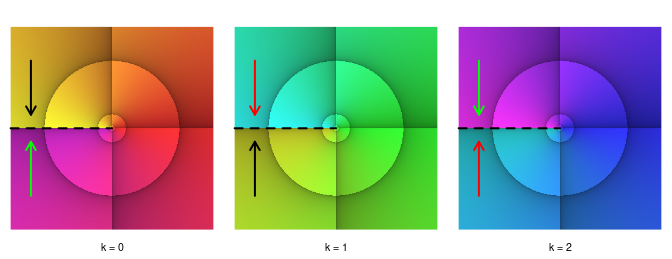Three phase portraits with branch cuts (dashed line), illustrating the three values of $$f(z)=z^{1/3}$$, $$z \in \mathbb{C} \setminus \lbrace 0 \rbrace$$. The transitions between the phase portraits are indicated by same-coloured arrows pointing at the branch cuts.

First, have a look at the leftmost diagram. Note that the argument reference lines have been adjusted to represent angle distances of $$30°$$, i.e. pi2Div = 12. Most noticeable is the abrupt color change from yellow to magenta along the negative real axis (emphasized with a dashed line). This is what is called a branch cut, and it suggests that our picture of the function $$f(z)=z^{1/3}$$ is not complete. As the three third roots of any complex number $$z=r\cdot\mathrm{e}^{\mathrm{i}\varphi}, z\in\mathbb{C}\setminus\lbrace0\rbrace$$ are $$r^{1/3}\cdot\mathrm{e}^{\mathrm{i}\cdot(\varphi+k\cdot2\pi)/3}; k=0,1,2; \varphi\in\left[0,2\pi\right[$$, we require three different phase portraits, one for each $$k$$, as shown in the figure above. With the argument reference line distance being $$30°$$, it is easy to see that each phase portrait covers a total argument range of $$120°$$, i.e. $$2\pi/3$$.

Obviously, each of the three portraits has a branch cut along the negative real axis, and the colors at the branch cuts show, where the transitions between the phase portraits have to happen. In the figure, we have illustrated this by arrows pointing to the branch cuts. Same-colored arrows in different phase portraits indicate the transitions. Thus, the first phase portrait ($$k = 0$$) links to the second ($$k = 1$$) in their yellow zones (black arrows); the second links to the third ($$k = 2$$) in their blue zones (red arrows), and the third links back to the first in their magenta zones (green arrows). Actually, one could imagine to stack the three face portraits in ascending order, cut them at the dashed line, and glue the branch cuts together according to the correct transitions. The resulting object is a Riemann surface with each phase portrait being a ‘sheet’. See more about this fascinating concept in Wegert (2012), Chapter7.

While the function $$f(z)=z^{1/3}$$ could be fully covered with three phase portraits, $$f(z)=\log z$$ has an infinite number of branches. As the (natural) logarithm of any complex number $$z=r\cdot\mathrm{e}^{i\cdot\varphi}, r>0$$ is $$\log z=\log r+\mathrm{i}\cdot\varphi$$, it is evident that the imaginary part of $$\log z$$ increases linearly with the argument of $$z$$, $$\varphi$$. In terms of phase portraits, this means an infinite number of stacked ‘sheets’ in either direction, clockwise and counterclockwise. Neighboring sheets connect at a branch cut. We illustrate this below with a phase portrait of $$\log z=\log r+\mathrm{i}\cdot(\varphi+k\cdot2\pi), r > 0, \varphi\in\left[0,2\pi\right[$$ for $$k=-1, 0, 1$$.

op <- par(mfrow = c(1, 3), mar = c(0.4, 0.2, 0.2, 0.2))
for(k in -1:1) {
FUNstring <- paste0("log(Mod(z)) + 1i * (Arg(z) + 2 * pi * ", k, ")")
phasePortrait(FUN = FUNstring, pi2Div = 36,
xlim = c(-2, 2), ylim = c(-2, 2), axes = FALSE, nCores = 2)
segments(-2, 0, 0, 0, col = "white", lwd = 1, lty = "dashed")
title(sub = paste0("k = ", k), line = -1)
}
par(op)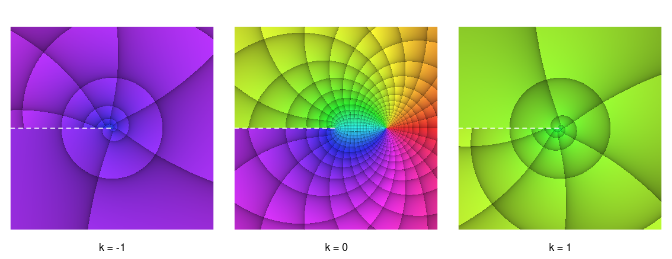Three branches of $$\log z=\log r+\mathrm{i}\cdot(\varphi + k\cdot2\pi), r>0, \varphi\in\left[0,2\pi\right[$$, with $$k=-1,0,1$$. The branch cuts are marked with dashed white lines.

### Riemann sphere plots

A convenient way to visualize the whole complex number plane is based on a stereographic projection suggested by Bernhard Riemann (see Wegert (2012), p. 20 ff. and p. 39 ff.). The Riemann Sphere is a sphere with radius 1, centered around the origin of the complex plane. It is cut into an upper (northern) and lower (southern) half by the complex plane. By connecting any point on the complex plane to the north pole with a straight line, the line’s intersection with the sphere’s surface marks the location on the sphere where the point is projected onto. Thus, all points inside the unit disk on the complex plane are projected onto the southern hemisphere, the origin being represented by the south pole. In contrast, all points outside the unit disk are projected onto the northern hemisphere, the north pole representing the point at infinity. For visualizing both hemispheres as 2D phase portraits, they have to be projected onto a flat surface in turn.

If we perform a stereographic projection of the southern hemisphere from the north pole to the complex plane (and look at the plane’s upper - the northern - side), this obviously results in a phase portrait on the untransformed complex plane as were all examples shown so far in this text. We can perform an analogue procedure for the northern hemisphere, projecting it from the south pole to the complex plane. We now want to think of the northern hemisphere projection as layered on top of the southern hemisphere projection, for the northern hemisphere, which it depicts, is naturally also on top of the southern hemisphere. If, in a ‘normal’ visualization of the complex plane (orthogonal real and imaginary axes), a point at any location represents a complex number $$z$$, a point at the same location in the northern hemisphere projection is mapped into $$1/z$$. The origin is mapped into the point at infinity. Technically, this mapping can be easily achieved when calling the function phasePortrait by setting the flag invertFlip = TRUE (default is FALSE). The resulting map is, in addition, rotated counter-clockwise around the point at infinity by an angle of $$\pi$$. As Wegert (2012) argues, this way of mapping has a convenient visual effect: Consider two phase portraits of the same function, one made with invertFlip = FALSE and the other one with invertFlip = TRUE. Both are shown side by side (see the pairs of phase portraits in the next two figures below). This can be imagined as a view into a Riemann sphere that has been cut open along the equator and swung open along a hinge in the line $$\Re(z)=1$$ (if the southern hemisphere is at the left side) or $$\Re(z)=-1$$ (if the northern hemisphere is at the left side). In order to highlight the Riemann sphere in Phase Portraits if desired, we provide the function riemannMask. Let’s first demonstrate this for the function $$f(z)=z$$.

op <- par(mfrow = c(1, 2), mar = rep(0.1, 4))
# Southern hemisphere
phasePortrait("z", xlim = c(-1.4, 1.4), ylim = c(-1.4, 1.4),
pi2Div = 12, axes = FALSE, nCores = 2)
riemannMask(annotSouth = TRUE)
# Northern hemisphere
phasePortrait("z", xlim = c(-1.4, 1.4), ylim = c(-1.4, 1.4),
pi2Div = 12, axes = FALSE, invertFlip = TRUE, nCores = 2)
riemannMask(annotNorth = TRUE)
par(op)